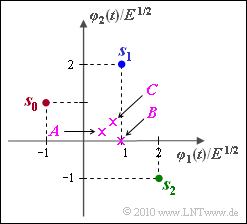# Exercise 4.08: Decision Regions at Three SymbolsSignal space constellations with  $M = 3$  symbols

We consider a signal space constellation in the two-dimensional space  $(N = 2)$  with the signal set:

$$\boldsymbol{ s }_0 = (-1, 1)\hspace{0.05cm}, \hspace{0.2cm} \boldsymbol{ s }_1 = (1, 2)\hspace{0.05cm}, \hspace{0.2cm} \boldsymbol{ s }_2 = (2, -1)\hspace{0.05cm},$$

in each case referred to the normalization value  $\sqrt {E}$.

The decision regions  $I_0$,  $I_1$  and  $I_2$ are sought,  with the following considerations:

1. The region  $I_i$  should contain the signal space point  $\boldsymbol{s}_i$   ($i = 0,\ 1,\ 2$).
2. The signals  $\boldsymbol{s}_0$,  $\boldsymbol{s}_1$  and  $\boldsymbol{s}_2$  are equally probable.
3. The regions are to be determined in such a way that the smallest error probability results for the AWGN channel.

With these preconditions,  the decision boundaries  $G_{\it ik}$  between regions  $I_i$  and  $I_k$  are respectively straight lines exactly midway between  $\boldsymbol{s}_i$  and  $\boldsymbol{s}_k$   $(i = 0,\ 1,\ 2; \ \ k = 0,\ 1,\ 2; \ \ i ≠ k)$.

With crosses in the above graph are three received values

$$\boldsymbol{ A } = (0.50, \hspace{0.1cm}0.25)\hspace{0.05cm}, \hspace{0.2cm} \boldsymbol{ B } = (1, \hspace{0.1cm}0)\hspace{0.05cm}, \hspace{0.2cm} \boldsymbol{ C } = (0.75, \hspace{0.1cm}0.50)$$

are drawn,nbsp; each of which is to be assigned to a region $I_i$  in subtask  (5).

Notes:

• To simplify the notation, the following is used:
$$x = {\varphi_1(t)}/{\sqrt{E}}\hspace{0.05cm}, \hspace{0.2cm} y = {\varphi_2(t)}/{\sqrt{E}}\hspace{0.05cm}.$$

### Questions

1

What is the equation of the decision boundary  $G_{\rm 01}$?

 $y = 3/2 \, -2 \cdot x$, $y = x/3$, $y = \, -3/4 + 3/2 \cdot x$.

2

What is the equation of the decision boundary  $G_{\rm 02}$?

 $y = 3/2 \, -2 \cdot x$, $y = x/3$, $y = \, -3/4 + 3/2 \cdot x$.

3

What is the equation of the decision boundary  $G_{\rm 12}$?

 $y = 3/2 \, -2 \cdot x$, $y = x/3$, $y = \, -3/4 + 3/2 \cdot x$.

4

Sketch the three decision regions  $I_0$,  $I_1$  and  $I_2$.  Do the decision boundaries  $G_{\rm 01}$,  $G_{\rm 02}$  and  $G_{\rm 12}$  intersect at a point?

 Yes. No.

5

Which of the following decisions are correct?

 $\boldsymbol{A} = (0.5,\ 0.25)$  belongs to region  $I_0$. $\boldsymbol{B} = (1,\ 0)$  belongs to region  $I_2$. $\boldsymbol{C} = (0.75,\ 0.5)$  belongs to region  $I_1$.

### Solution

#### Solution

(1)  Solution 1  is correct:

• The connecting line between the signal points  $\boldsymbol{s}_0 = (–1,\ 1)$  and  $\boldsymbol{s}_1 = (1,\ 2)$  has the gradient  $1/2$  (see diagram).
• The decision boundary intersects the connecting line at  $(\boldsymbol{s}_0 + \boldsymbol{s}_1)/2 = (0,\ 1.5)$  and has the gradient  $2$  $($rotation of the connecting line by  $90^\circ)$.
• From this follows:   $y = 1.5 - 2 x \hspace{0.05cm}.$

(2)  Solution 3  is correct:

• The connecting line between  $\boldsymbol{s}_0 = (–1,\ 1)$  and  $\boldsymbol{s}_2 = (2,\ 1)$  has the gradient  $–2/3$  and intersects the decision boundary  $G_{\rm 02}$  $($with gradient $3/2)$  at $(0.5,\ 0)$.
• From this follows:   $y = {3}/{2} \left ( x - {1}/{2} \right ) = -{3}/{4} + {3}/{2} \cdot x\hspace{0.05cm}.$

(3)  Here  solution 2  is applicable:

• The line connecting  $\boldsymbol{s}_1 = (1,\ 2)$  and  $\boldsymbol{s}_2 = (2, \, –1)$  intersects the decision boundary  $G_{\rm 12}$  at  $(1.5,\ 0.5)$  and has gradient  $–3$.
• Consequently,  the gradient of  $G_{\rm 12} = 1/3$  and the equation of the decision boundary $G_{\rm 12}$ is:
$$y - {1}/{2} = {1}/{3} \cdot \left ( x - {3}/{2} \right ) = {x}/{3} - {1}/{2}\hspace{0.3cm}\Rightarrow \hspace{0.3cm}y = {x}/{3} \hspace{0.05cm}.$$

• The intersection of  $G_{\rm 01}$  and  $G_{\rm 12}$  (white circle)  is at  $(9/14,\ 3/14)$,  because of
$${3}/{2} - 2 x = {x}/{3} \hspace{0.3cm}\Rightarrow \hspace{0.3cm} {3}/{2} = {7}/{3} \cdot x \hspace{0.3cm} \Rightarrow \hspace{0.3cm} y = {3}/{14} \hspace{0.05cm}.$$
• The straight line  $G_{\rm 02}$  also passes through this point:
$$y(x = {9}/{14}) =-{3}/{4} + {3}/{2} \cdot x = -{3}/{4} + {3}/{2} \cdot {9}/{14} =\frac{-21+27}{28}= {3}/{14} \hspace{0.05cm}.$$